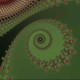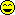January 10, 2012 by jeffspc88mxIn the last bit of the dipswitch project code, I'm writing the values of the 2 "bytes" (3-bit bytes, I know) to the LCD with: ``````lcd_write_string(PSTR("a: ")); lcd_write_int16(a1*1 + a2*2 + a3*4); lcd_write_string(PSTR("; b: ")); lcd_write_int16(b1*1 + b2*2 + b3*4); `````` ...and that works, but when I try to add these two expressions with... ``````lcd_line_two(); lcd_write_string(PSTR("SUM: ")); lcd_write_int16(a1*1 + a2*2 + a3*4 + b1*1 + b2*2 + b3*4); `````` ...I get various nonsense answers, and always a leading zero, and never "00" - always some positive non-zero number. I'm a C novice, but a VBA journeyman; is my syntax wrong for C? Try: ``````lcd_write_int16((uint16_t)(a1*1 + a2*2 + a3*4 + b1*1 + b2*2 + b3*4)); `````` It may be a casting problem. Hi pcbolt, I have a theory, try adding this line ``````lcd_write_string(PSTR(" ")); `````` right after this line ``````lcd_write_int16(a1*1 + a2*2 + a3*4 + b1*1 + b2*2 + b3*4); `````` and let me know what happens. Hi Humberto - Think you want jeffspc88mx. Glad to get a response, tho =)2 pts. for Humberto! 'Twas the trailing digit. After generating a two digit answer, it wasn't erasing the second digit when it was time to report a 1 digit answer. So... ``````7+1 = 8 7+3 = 10 3+3 = 60 1+3 = 40 `````` and so on. Forcing it to write some space after the answer wipes the trailing zero.